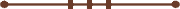####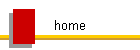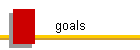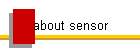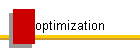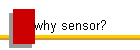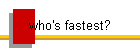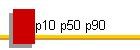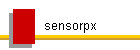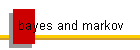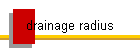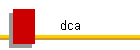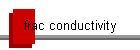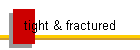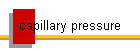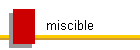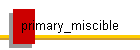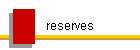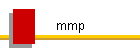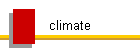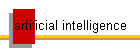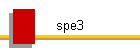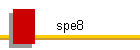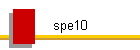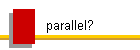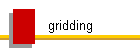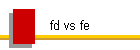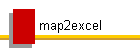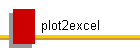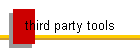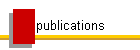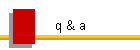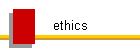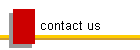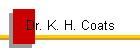### Fracture Conductivity and (or) Permeability, Width, and Transmissibility

Fracture conductivity, defined as fracture permeability times width kf w, is a measure of how easily fluids flow through a fracture. Absolute fluid flow rate between fractures in two adjacent gridblocks of given sizes is proportional to its conductivity, but flux is (a far more useful measure of fracture flow and is) proportional to permeability (and potential gradient and fluid mobility).

Relative change in fracture conductivity due to fracturing, propping, and closure  is a useful measure, as it is equal to the relative change in numerical model transmissibilties (or transmissibility modifiers) that should be applied  due to the resulting effects on both fracture permeability and width.

Flow rates and fluxes in fractures (and matrix)  are proportional to permeability.  Permeability is a physical property of porous systems and fractures, equal to the ratio of  volumetric flux to the potential gradient for a unit-mobility fluid.  Permeability times area (not fracture width), is equal to the ratio of volumetric flow rate to the potential gradient for a unit-mobility fluid across a given area in the direction of flow.

In the limit as fracture width (or matrix porosity) approaches 0, fracture permeability (or matrix permeability) also approaches 0.  Permeability can be measured in the lab and theoretically predicted, and is therefore usually given as input data (along with fracture width) to our numerical models of fractured and unfractured reservoirs, rather than conductivity.  Our reservoir models incorporate both permeability (or conductivity for fracture blocks if it were desired) and fracture width into "transmissibility" terms that are usually constant in conventional reservoirs.  Numerical model transmissibilities are proportional to conductivity.  Field units of transmissibilty are rb cp / (psia d).  They represent the ratio of the volumetric rate of flow to the potential difference between each pair of connected gridblocks for a unit-mobility fluid.

Consider 1D x-direction flow in a horizontal (xy) fracture from fracture block 1 to fracture block 2 with fixed fracture block dimensions dx, dy, and dz = fracture width w (ft), ignoring effects of gravity and capillary pressure.

1                                   2

 A = w dy

L = dx                                w = dz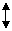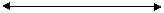kf = fracture permeability = constant (md)

Cf = fracture conductivity = kf  w = constant (md ft)

The discretized multiphase form of Darcy's law (assuming laminar flow) gives the volumetric flow rate of phase j from block 1 to block 2 as:

qj = (.001127 kf A / L)   λj (P1 - P2)   =  Tx  λj (P1 - P2)  (rb/d), or

qj = (.001127 Cf dy / L) λj (P1 - P2)   =  Tx  λj (P1 - P2)  (rb/d)

where λj is the phase mobility = relative permeability / viscosity = krj / vj, and Tx is the x-direction transmissibility between blocks 1 and 2.  Transmissibility is a function of gridblock dimensions dx,dy,dz (w) and permeability, or conductivity.  Turbulent flow can be modeled in the well flow terms but usually can be neglected for block-to-block flow inside the reservoirs, especially when the infinite permeability assumption applies (all turbulence can be neglected in that case, as increasing well PI or fracture permeability has no effect on results).

The volumetric flux is

qj* = qj / A = (.001127 kf) λj [ (P1 - P2) / L ]  (rb/ft2/d)

The last term in brackets is the numerical approximation of potential gradient.  So,

kf = ratio of volumetric flux to the potential gradient for a unit-mobility fluid.

Flux in a fracture is independent of w, except as it may effect the value of permeability.

kf A = ratio of flow rate to the potential gradient for a unit-mobility fluid.  A is the area in the direction of flow.

So, the most appropriate term characterizing fracture flow rate is kf A, not Cf=kf w.

Both permeability (or conductivity) and fracture width affect fracture flow rate, and both are represented along with gridblock geometry in the numerical model transmissibilities.

The flux written in terms of conductivity is

qj* = qj / A = (.001127 Cf / w) λj [ (P1 - P2) / L ]  (rb/ft2/d)

with w in ft.  So if we wish we can address ideal fracture flow capacity in terms of the ratio of conductivity to width (equals permeability), and the effects of fracturing, propping, and closure on both of those variables, or we can simply address those effects on the basic meaningful variables, permeability and width, and on the resulting numerical model transmissibilities that reflect both of them.

Transmissibility T = 0.001127 k A / L = ratio of volumetric flow rate to the potential difference between two gridblocks across area A  (= w dy) for a unit-mobility fluid.  It is usually considered constant for unfractured and for naturally fractured reservoirs (no stress dependence of either matrix or fracture permeability) unless extreme pressure is applied, as in hydraulic fracturing.

The simulator will predict the correct flow rate and flux if dx is small enough to prevent significant error (numerical dispersion) due to numerical finite-difference approximation of the pressure gradient.  Permeability is a meaningful measure of fracture flow capacity as a function of pressure gradient.  Conductivity by itself is not (unless divided by w to obtain permeability).  That is why we measure permeability in the lab, rather than conductivity.

Dimensionless fracture conductivity is usually referred to as Fcd=kfw/(kmxf), conductivity divided by matrix permeability times fracture half length.  None of those parameters are a constant in any real naturally and/or hydraulically fractured system.  But for the ideal homogeneous and isotropic single ideal planar fracture in matrix, Fcd is equivalent to kfAf/(kmAmf), which indicates the ratio of fracture to matrix flow for identical pressure gradients and fluid mobility.  Af is the fracture area in direction of flow along the fracture, and Amf is the area of contact between matrix and fracture.

All flows are functions of pressure gradient distributions  (including gravity and capillary forces) and fluid mobilities in matrix and fracture, and flow from matrix to fracture is additionally a function of matrix permeability and the area in contact with fractures., while flow rates along fractures are additionally a function of fracture permeability and  area in direction of flow.

The simple ratio of permeabilities has physical meaning as the ratio of flux through the fractures to that through the matrix for a given pressure gradient and fluid. But that doesn't mean much. and neither does Fcd, since potential gradients are very large in tight matrix and very small in fractures.  In general, all of the governing equations must be solved in a reservoir simulator to make any conclusions of how flow through matrix and fractures and production will behave as functions of the input variables (distributions of permeability and porosity and rocktype/relperm/Pc, pvt, initial and boundary conditions, frac models, ...).  Those conclusions will generally be case-dependent.  No single dimensionless quantity can possibly characterize the description or behavior of any heterogeneous system.

The "infinite conductivity" assumption or level is equivalent to the "infinite permeability" assumption that we may check with our reservoir models.  That means that the conductivity or permeability (for given fracture distributions and assumed behavior) is high enough such that the pressure drop in the fractures is negligible.  This is the solution that should always be found first.  Then, sensitivities to reduced values of fracture permeability, along with the effects of any assumptions or representations regarding fracture dimensional or volumetric behavior and distributions, can be examined with the simulator.

For an open planar fracture of width w (in), permeability is1:

kf = 54 * 109 w**2 (md)

and the flux of phase j through an ideal open fracture is

qj* = .001127 kf λj [ (P1 - P2) / L ] = 6.0858 * 107 w**2 λj [ (P1 - P2) / L ]    (rb/ft2/d)

If a fracture is packed with proppant, then the fracture permeability will be equal to that of the packed proppant bed, if particle size is small compared to w.

If the proppant is crushed or degrades over time due to increased stress as pressure falls below fracture pressure during production, then w will change and that will have the indicated effect on numerical model fracture gridblock transmissibility (Tx =  k w dy / L).  Permeability of the packed proppant may or will also decrease due to these effects and will also have the indicated effect on fracture gridblock transmissibilities. Any relative decrease in permeability or conductivity or w causes flow rate and flux to decrease by the same relative amount, for a given potential gradient.

In a dual porosity or a dual permeability model, input values of fracture permeability are effective values, scaled to apply over the entire area of the dual porosity gridblock Ad. The effective dual fracture perm kxf* is equal to kxf Af / Ad, where Af / Ad is the ratio of the total fracture area to total area in the x-direction.  Fractures are represented in the dual gridblock by their spacings in the x,y, and z directions as Lx, Ly, and Lz.  These are affected or caused by fracturing, but do not change with stress and closure below fracture pressure.

Dual matrix-fracture exchange transmissibilities are not a function of fracture permeability or fracture width.  They depend only on matrix permeability and fracture spacings (See equation given for Tex in the Sensor Manual).

So how can we compute theoretically optimal well and fracture spacings in tight systems?  Sensor has several methods and options of varying complexity.

The simplest and fastest method is to assume constant identical well and fracture spacings LW and LX for areally parallel and optionally stacked horizontal wells, orthogonal planar hydraulic fractures of known geometry, frictionless wellbores, identical operation of all wells (boundary conditions) in a field-wide production strategy, and to include an economic model so that input parameters LW, LX, and Tend can be easily adjusted to maximize NPV, either by manual adjustment or by application of an optimization program.  Sensor's HWELEMENT and ECONOMICS options provide the ability to quickly determine optimal well and fracture spacings (and optionally generalized operational strategy including times and constraints for depletion, water and gas  or wag flooding, and blowdown*), and examine their dependencies for idealized systems. The assumptions allow the use of a horizontal well pattern element for simulation of interior well performance, minimizing model size and runtime and the number of variables, and maximizing our abilities in iterative predictive optimization, and even allow probabilistic optimizations and predictions using advanced workflows, given uncertainties in the Sensor input data and/or represented in an arbitrary number of equally-probable realizations of the uncertainties (Sensor datafiles) from any source.  The size of the grid is determined by the user to control numerical dispersion in determining the correct fine-grid solution without the need for upscaling (the grid is refined until there is no significant change in results).  Any heterogeneities may be included but they are symmetrical across planes of symmetry.  They may be specified using probability distributions in the Sensor data for the probabilistic workflows, which are randomly populated at the time of execution  The pattern element method otherwise applies to layered systems with reservoir properties that are constant by layer. See SensorFrac_HWE.dat for an example.

The discrete hydraulic fractures of known size can be replaced by Sensor's dual porosity or dual permeability empirical Fracture Model that predicts fracture generation, dilation, propagation, propping, and closure.  That model can also be applied to any existing natural fracture network that may be initially almost sealed, and/or to the matrix to represent stimulation below fracture pressure.  Sensor provides the ability to simulate (optimize, match and predict) the hydraulic fracturing and production processes in a single model, with or without the pattern element option, natural fractures, matrix or natural fracture stimulation, data uncertainties, or existing workflows for automated upscaling, history matching, optimizations, and predictions using third-party optimization software.

Pipe-it® workflow integration and optimization software was developed by our associate Curtis Whitson and his team at Petrostreamz (now owned by Tieto).  When used with an applicable predictive model, Pipe-it® has provided for the general solution of the automatic predictive optimization problem.  Any number of applications may be linked together to form workflows.  Any input data may be iteratively and automatically adjusted using any of a number of available optimizers to maximize or minimize any objective function definable by the workflow outputs.  We have developed workflows within Pipe-it® for Sensor that solve the probabilistic automatic predictive optimization problem, which considers uncertainties in the input data to produce probabilistic results.

Other vendors have also applied various optimization methods to Sensor to provide advanced workflows for assisted history matching, optimization, and forecasting (see our Third Party Tools page).  These deterministic and probabilistic workflows, using models of varying complexity, are now able to answer the questions that reservoir engineers have always asked, where should we put our wells and how should we complete and operate them, to minimize the cost of energy production?  The key to making them work well is to minimize model size and the number of optimization variables.  Pattern element or highly upscaled field models are ideal.

1 Aguilera, R., Naturally Fractured Reservoirs, PennWell Books, 1995, p.16

* Requires a compositional model.  Automatic iterative optimizers provide a vast step-change in our capabilities in achieving automatic generalized predictive optimization, versus manual optimization, when the number of optimization variables exceeds about two.

© 2000 - 2022 Coats Engineering, Inc.